# Math > Year 2

## Properties of 2D shapes

2D Shapes

A 2D (two-dimensional) shape is a flat shape that has only two dimensions.

2D shapes have sides and vertices. A vertex is a point where two or more lines meet. The plural of vertex is vertices.

Properties of a shape are the number of sides and vertices of a shape.

Let’s learn about the properties of different 2-D shapes.

Triangle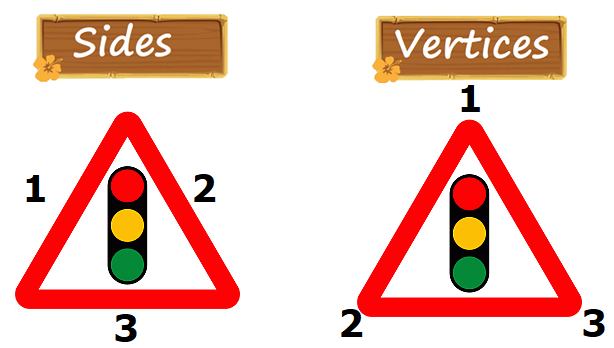A triangle has 3 sides and 3 vertices.

Square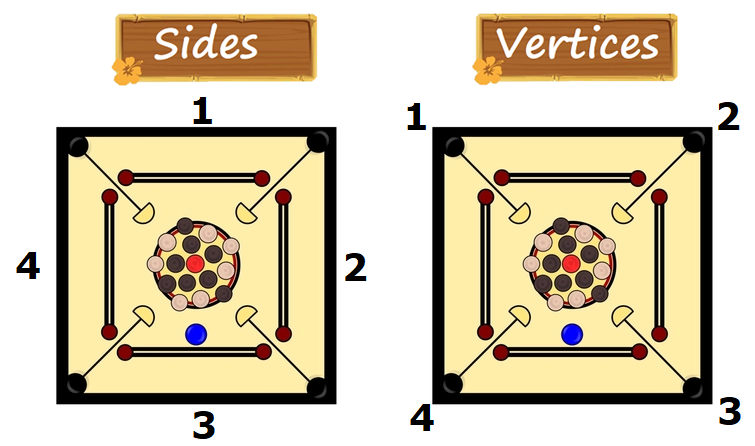A square has 4 sides and 4 vertices.

Rectangle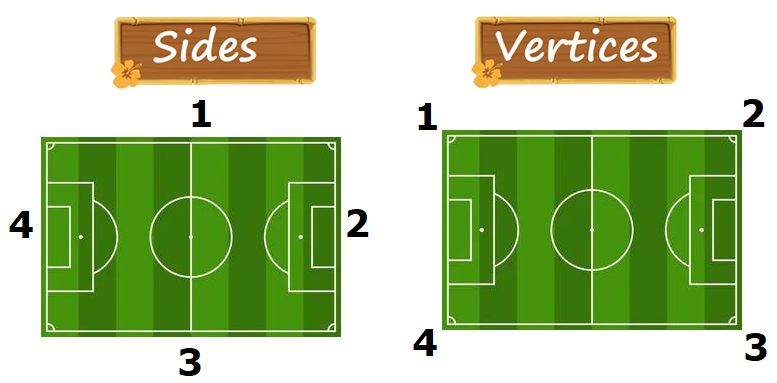A rectangle has 4 sides and 4 vertices.

Pentagon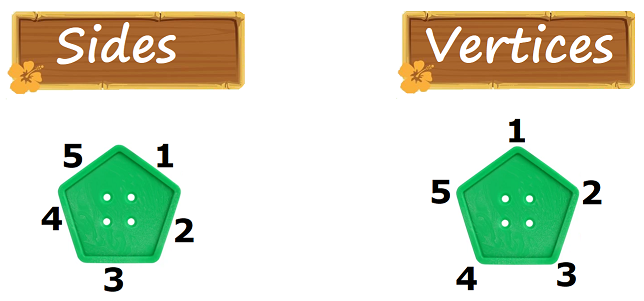Hexagon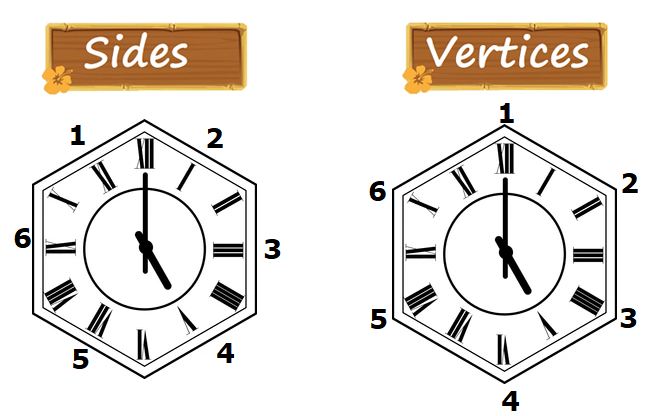A hexagon has 6 sides and 6 vertices.

Octagon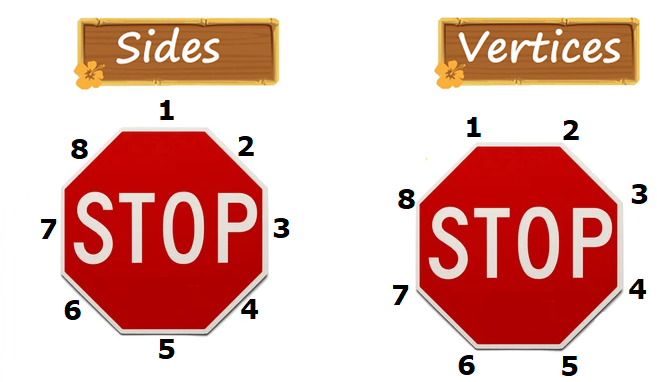An octagon has 8 sides and 8 vertices.

//